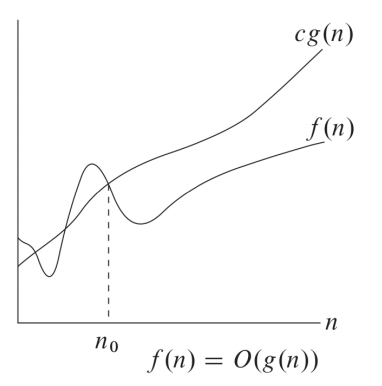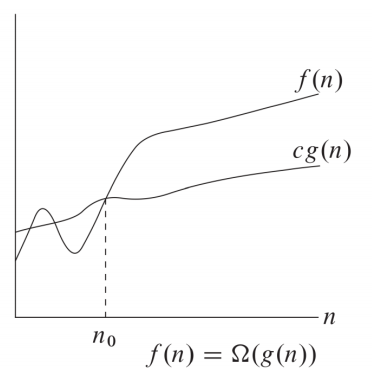# 1.渐进表示法

• \Omicron表示法

\Omicron (g(n)) = \{f(n): 存在正数c和n_0，使得当n \ge n_0时0 \le f(n) \le cg(n)恒成立 \}• \Omega表示法

\Omega (g(n)) = {f(n): 存在正数c和n_0，使得当n \ge n_0时0 \le cg(n) \le f(n)恒成立 }